# Prototyping a walking pattern generator

Walking trajectory generation is the problem of computing an ideal locomotion trajectory, sometimes called a walking pattern. It is the first sub-problem in the traditional walking control scheme for legged robots. A walking trajectory is dynamically consistent (it respects the laws of physics) and unperturbed (it assumes all controls are perfectly executed by the robot). In this post, we will see how to design a complete walking trajectory generator in pymanoid using polynomial interpolation for the swing leg and model predictive control for the center of mass.

## Footstep planning

The goal of our walking trajectory generator will be to make the robot walk through a given sequence of contacts (in Python, a list of pymanoid.Contact objects). For walking forward, we can generate this sequence simply by placing footsteps to the left and right of a line segment:

import pymanoid

def generate_footsteps(distance, step_length, foot_spread):
contacts = []

def append_contact(x, y):
contacts.append(pymanoid.Contact(
shape=(0.11, 0.05), pos=[x, y, 0.], friction=0.7))

x = 0.
while x < distance:
if distance - x <= step_length:
x += min(distance - x, 0.5 * step_length)
else:  # still some way to go
x += step_length
y = -y
append_contact(x, y)
append_contact(x, -y)  # now x == distance
return contacts


The function depends on three parameters: distance, the total distance in meters to walk, step_length, which is by definition the distance between right and left heel in double support, and the lateral distance foot_spread between left and right foot centers. The first and last steps have half step length so as to accelerate and decelerate more progressively. In what follows, the robot will walk seven steps forward with a step length of 30 cm:

footsteps = generate_footsteps(
distance=2.1,
step_length=0.3,
friction=0.7)


The robot starts from a standing posture with its center of mass (COM) 85 cm above ground, which is a comfortable height for the default JVRC-1 model. We put the robot in this initial posture by solving the inverse kinematics (IK) of our desired stance (a pymanoid.Stance):

if __name__ == "__main__":
dt = 0.03  # simulation time step, in [s]
sim = pymanoid.Simulation(dt=dt)
sim.set_viewer()
stance = pymanoid.Stance(
com=pymanoid.PointMass([0, 0, 0.85], robot.mass),
left_foot=footsteps,
right_foot=footsteps)
stance.bind(robot)
robot.ik.solve(max_it=42)


Our robot is now ready to walk: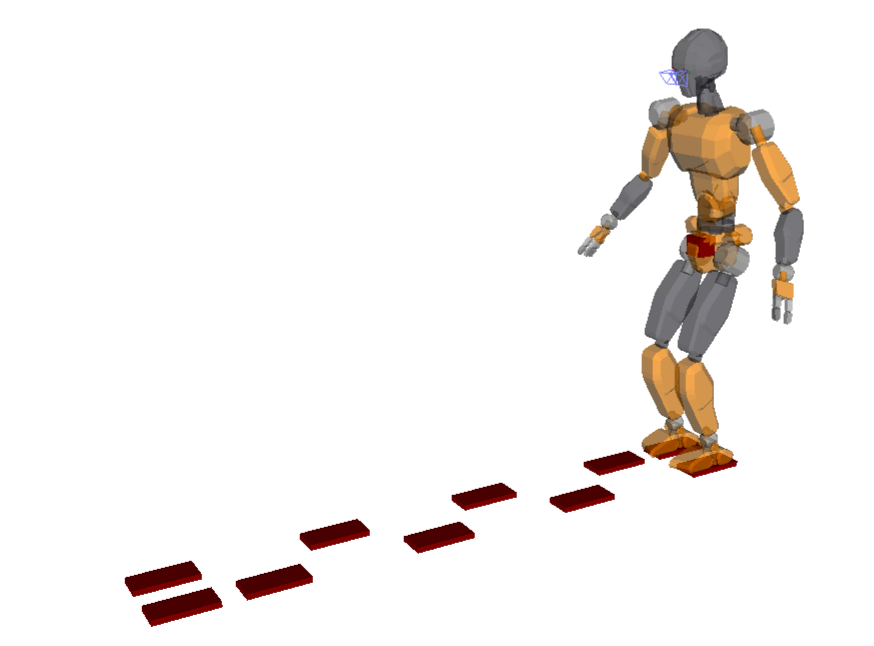## Walking state machine

Walking alternates double support phases (DSP), where both feet are in contact with the ground, and single support phases (SSP), where one foot (the swing foot) is in the air while the robot supports its weight on the other one: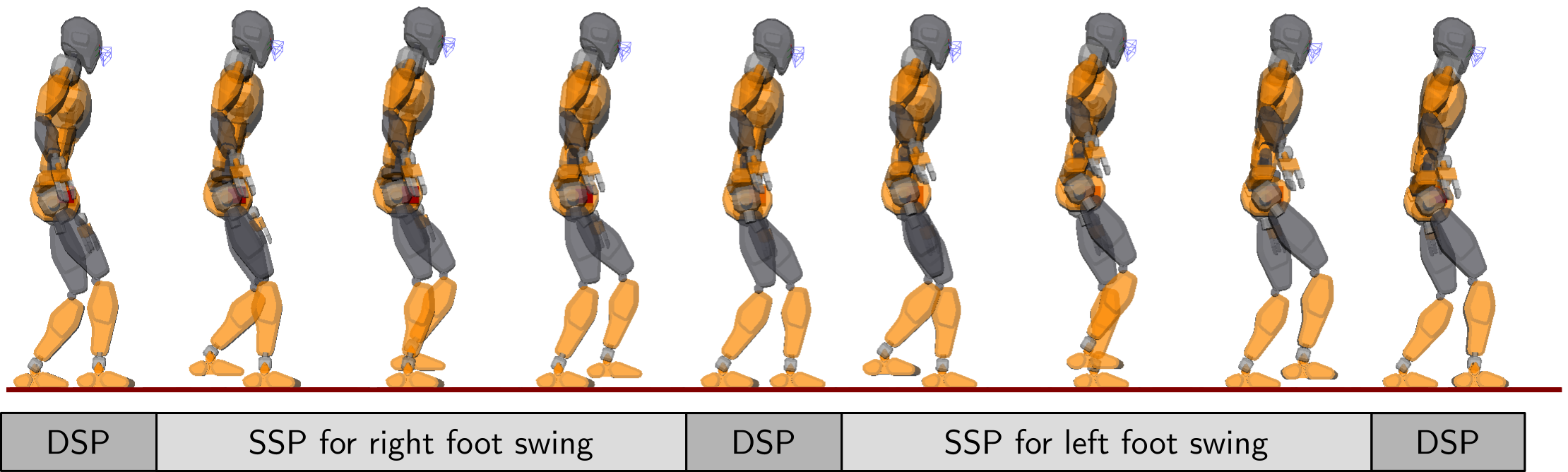We can represent these phases in a finite-state machine (FSM) with three states, standing, double support and single support, implemented as a pymanoid.Process:

class WalkingFSM(pymanoid.Process):

def __init__(self, ssp_duration, dsp_duration):
super(WalkingFSM, self).__init__()
self.dsp_duration = dsp_duration
self.mpc_timestep = 3 * dt  # update MPC every 90 [ms] (see below)
self.next_footstep = 2
self.ssp_duration = ssp_duration
self.state = None
#
self.start_standing()

def on_tick(self, sim):
if self.state == "Standing":
return self.run_standing()
elif self.state == "DoubleSupport":
return self.run_double_support()
elif self.state == "SingleSupport":
return self.run_single_support()
raise Exception("Unknown state: " + self.state)


The state machine will switch between single and double support phases based on the pre-defined phase timings ssp_duration and dsp_duration.

### Standing state

For each state, we define a "start" and "run" function. The standing state simply waits for the user to set the start_walking boolean attribute to True:

# class WalkingFSM(pymanoid.Process):

def start_standing(self):
self.start_walking = False
self.state = "Standing"
return self.run_standing()

def run_standing(self):
if self.start_walking:
self.start_walking = False
if self.next_footstep < len(footsteps):
return self.start_double_support()


### Double support state

For starters, the double support state simply waits for its phase duration, then switches to single support:

# class WalkingFSM(pymanoid.Process):

def start_double_support(self):
if self.next_footstep % 2 == 1:  # left foot swings
self.stance_foot = stance.right_foot
else:  # right foot swings
self.stance_foot = stance.left_foot
dsp_duration = self.dsp_duration
if self.next_footstep == 2 or self.next_footstep == len(footsteps) - 1:
# double support is a bit longer for the first and last steps
dsp_duration = 4 * self.dsp_duration
self.swing_target = footsteps[self.next_footstep]
self.rem_time = dsp_duration
self.state = "DoubleSupport"
self.start_com_mpc_dsp()
return self.run_double_support()

def run_double_support(self):
if self.rem_time <= 0.:
return self.start_single_support()
self.run_com_mpc()
self.rem_time -= dt


We use the start function of the state to save two important contact locations, namely the stance foot and swing target of the next SSP. The reason for saving them here rather than in the start function of the SSP itself will become clear later on, when we implement the start_com_mpc_dsp() and run_com_mpc() functions. For now, let us complete them with a dummy linear interpolation to debug the development of our ongoing FSM:

# class WalkingFSM(pymanoid.Process):

def start_com_mpc_dsp(self):
pass  # to be implemented later

def run_com_mpc(self):
stance.com.set_x(0.5 * (self.swing_foot.x + self.stance_foot.x))


### Single support state

The single support phase interpolates both COM and swing foot trajectories, and updates stance targets accordingly:

# class WalkingFSM(pymanoid.Process):

def start_single_support(self):
if self.next_footstep % 2 == 1:  # left foot swings
self.swing_foot = stance.free_contact('left_foot')
else:  # right foot swings
self.swing_foot = stance.free_contact('right_foot')
self.next_footstep += 1
self.rem_time = self.ssp_duration
self.state = "SingleSupport"
self.start_swing_foot()
self.start_com_mpc_ssp()
self.run_single_support()

def run_single_support(self):
if self.rem_time <= 0.:
stance.set_contact(self.swing_foot)
if self.next_footstep < len(footsteps):
return self.start_double_support()
else:  # footstep sequence is over
return self.start_standing()
self.run_swing_foot()
self.run_com_mpc()
self.rem_time -= dt


For now, we apply dummy linear interpolations for both the COM and swing foot trajectories:

# class WalkingFSM(pymanoid.Process):

def start_swing_foot(self):
self.swing_start = self.swing_foot.pose

def start_com_mpc_ssp(self):
pass  # to be implemented later

def run_swing_foot(self):
progress = min(1., max(0., 1. - self.rem_time / self.ssp_duration))
new_pose = pymanoid.interp.interpolate_pose_linear(
self.swing_start,
self.swing_target.pose,
progress,
)
self.swing_foot.set_pose(new_pose)


When the phase time reaches the desired SSP duration, the state machine switches back to double support and the process repeats until the robot has traversed all footsteps in the sequence, finally switching back to the standing state.

### Scheduling simulation processes

Although we haven't implemented neither swing foot nor COM trajectories yet, we can already validate this code in simulation. Let us create an FSM process with phase durations roughly 0.7/0.1 seconds for single and double support respectively:

ssp_duration = 24 * dt  # 720 [ms]
dsp_duration = 3 * dt  # 90 [ms]
fsm = WalkingFSM(ssp_duration, dsp_duration)


As the FSM updates targets, we schedule it before inverse kinematics in the simulation:

sim.schedule(fsm)
sim.schedule(robot.ik)
sim.start()


Let us throw in some extra drawer processes to keep track of the COM and swing foot position targets while the robot moves:

com_traj_drawer = TrajectoryDrawer(robot.stance.com, 'b-')
lf_traj_drawer = TrajectoryDrawer(robot.left_foot, 'g-')
rf_traj_drawer = TrajectoryDrawer(robot.right_foot, 'r-')
sim.schedule_extra(com_traj_drawer)
sim.schedule_extra(lf_traj_drawer)
sim.schedule_extra(rf_traj_drawer)


Now, the only thing left to do to start walking is:

In : fsm.start_walking = True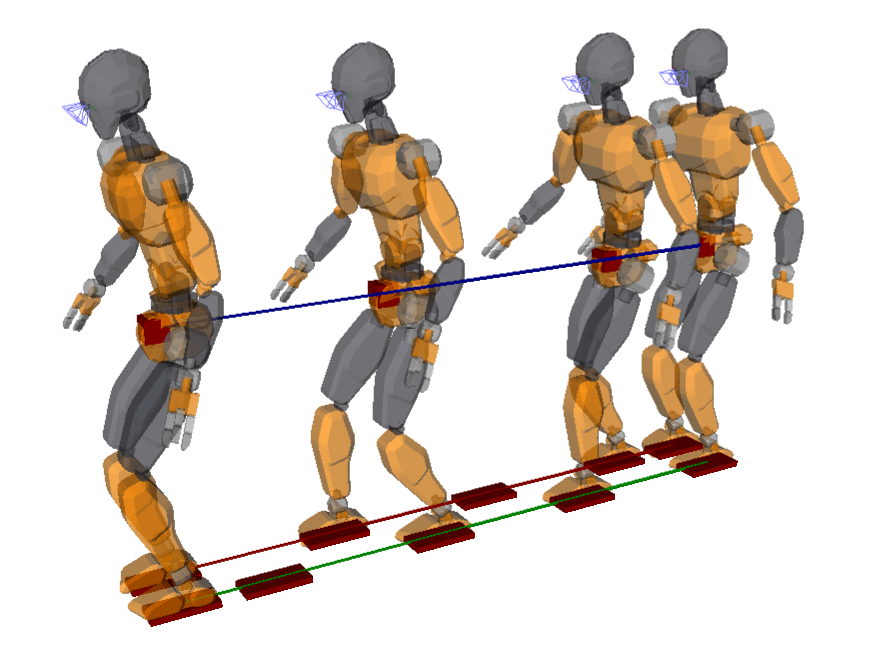The robot shuffles its feet in a motion that is kinematically but not dynamically consistent. We can see that by scheduling an extra wrench drawer to check the dynamics of the simulation:

wrench_drawer = PointMassWrenchDrawer(stance.com, stance)
sim.schedule_extra(wrench_drawer)


This process draws feasible contact forces when it finds some, otherwise it colors the background in red to show that the motion is not dynamically feasible. This is what happens most of the time with our controller at this point.

## Swing foot interpolation

Our fixed footstep sequence provides initial and target configurations of the swing foot for each SSP. For this first prototype, let us implement a "flat foot" walk where the swing foot stays parallel to the ground. This strategy is easier to implement, but on real robots it has a tendency to yield early contacts and larger impacts than the heel-strike toe-off walk commonly applied by humans.

The orientation of the swing foot being given, the main parameter to tune is the vertical clearance of the trajectory. The default pymanoid.SwingFoot interpolates a cubic Hermite spline parameterized by a takeoff clearance height $$a$$ and a landing clearance height $$b$$: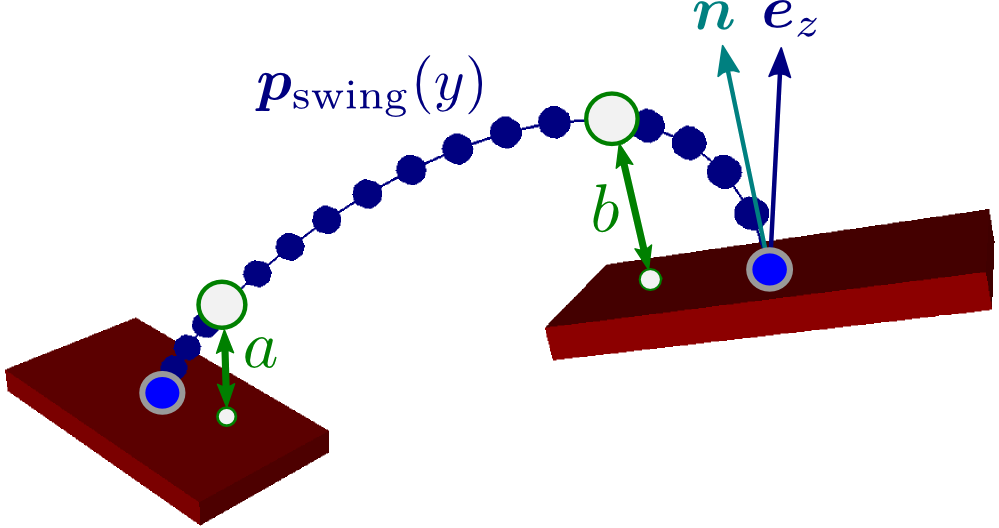For us the ground contact normal $$\bfn$$ coincides with the world vertical $$\bfe_z$$ in the figure above. The swing foot interpolator computes a polynomial $$P(t)$$ such that:

• $$P(0)$$ is the initial foot position,
• $$P(T_\textit{SSP})$$ is the target foot position, with $$T_\textit{SSP}$$ the duration of the single support phase,
• $$P(\frac{1}{4} T_\textit{SSP})$$ is at height greater than $$a$$ from the initial contact, and
• $$P(\frac{3}{4} T_\textit{SSP})$$ is at height greater than $$b$$ from the target contact.

We can choose $$a = b = 5~\text{cm}$$ so that the foot detaches neatly from the ground without causing too much vertical height variations of the COM. First, let us instantiate the interpolator by:

# class WalkingFSM(pymanoid.Process):

def start_swing_foot(self):
self.swing_start = self.swing_foot.pose
self.swing_interp = SwingFoot(
self.swing_foot, self.swing_target, ssp_duration,
takeoff_clearance=0.05, landing_clearance=0.05)


Second, we replace the dummy linear interpolation by the output from the swing foot interpolator:

# class WalkingFSM(pymanoid.Process):

def run_swing_foot(self):
new_pose = self.swing_interp.integrate(dt)
self.swing_foot.set_pose(new_pose)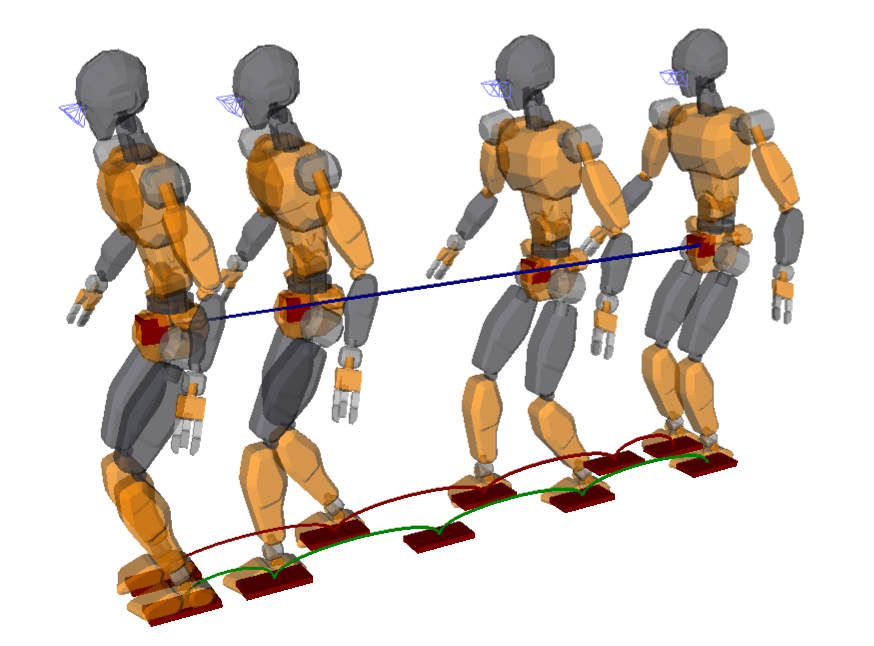Now the robot properly lifts its feet in a motion that is kinematically but still not dynamically consistent.

## Center of mass trajectory

The main challenge in walking trajectory generation is to make the motion dynamically consistent, that is, to make sure that the equations of motion

\begin{equation*} \bfM(\bfq) \qdd + \qd^\top \bfC(\bfq) \qd = \bfS^\top \bftau + \bftau_g(\bfq) + \sum_{i=1}^N \calJ_{C_i}^{\top} \bfw_{C_i} \end{equation*}

have physically feasible joint torques $$\bftau$$ and contact wrenches $$\bfw_{C_i} = [\bftau_{C_i}\ \bff_i]$$.

### Linear inverted pendulum mode

Assuming that the robot is powerful enough to execute the walking motion, we can omit the lines that contain $$\bftau$$ and focus on the Newton-Euler equations that govern the floating-base dynamics of the robot:

\begin{equation*} \begin{array}{rcl} m \bfpdd_G & = & m \bfg + \sum_i \bff_i \\ \dot{\bfL}_G & = & \sum_i (\bfp_{C_i} - \bfp_G) \times \bff_i + \bftau_{C_i} \end{array} \end{equation*}

For this first walking trajectory generator, we won't use rotations of the upper-body and focus on producing the linear horizontal motion of the center of mass. We rely for this purpose on the linear inverted pendulum model, keeping the COM at a constant height $$h$$ as well as a constant angular momentum $$\bfL_G = \boldsymbol{0}$$. As a consequence, the Euler equation reduces to having the resultant of contact forces going from the zero-tilting moment point (ZMP) to the COM, and the equation of motion becomes:

\begin{equation*} \bfpdd_G = \frac{g}{h} (\bfp_G - \bfp_Z) \end{equation*}

where $$Z$$ denotes the ZMP.

### Feasibility of contact forces

We want to make sure that, at every time instant, there exists feasible contact wrenches $$\bfw_{C_i} = [\bftau_{C_i}\ \bff_i]$$ that lie in their wrench friction cone. In the linear inverted pendulum mode, this is equivalent to having the ZMP inside its ZMP support area. This area is a convex polytope projection in general, fortunately when walking on a flat floor with large friction it reduces to the convex hull $$\cal C$$ of ground contact points:

\begin{equation*} \bfp_Z = \bfp_G - \frac{h}{g} \bfpdd_G \in {\cal C} \end{equation*}

### Linear model predictive control

Walking involves falling forward during single support phases, which is instantaneously unstable and only becomes "stable" when considering the future of the system (for instance, the next double support phase where the biped may slow down to a stop if needed). As such, walking trajectory generation is commonly solved by model predictive control (MPC) where the COM trajectory is computed over a preview horizon of one or two steps. This approach was initially known as preview control and reformulated with inequality constraints as linear model predictive control.

Let us focus on lateral COM motions (sagittal motions can be derived similarly), and define the state of our system as $$\bfx = [y_G\ \dot{y}_G\ \ddot{y}_G]$$. The MPC problem is then to generate a trajectory $$y_G(t)$$ such that:

• at the current time $$t_0$$, $$\bfx(t_0)$$ is equal to the current state of the COM $$[y_G(t_0)\ \dot{y}_G(t_0)\ \ddot{y}_G(t_0)]$$;
• at all times, the ZMP $$y_\textit{min}(t) \leq y_Z(\bfx(t)) \leq y_\textit{max}(t)$$ where $$y_\textit{min}(t)$$ and $$y_\textit{max}(t)$$ define the edges of the support area at time $$t$$;
• at the end of the preview horizon $$t_f$$, $$\bfx(t_f)$$ is equal to a desired terminal state, for instance $$[y_f\ 0\ 0]$$ where the robot has stopped walking over the last footstep.

We define control input as the COM jerk $$u = \dddot{y}_G$$, and discretize our system as:

\begin{equation*} \bfx_{k+1} = \bfA \bfx_k + \bfB u_k \end{equation*}

where the state matrix $$\bfA$$ and control matrix $$\bfB$$ are defined by:

\begin{equation*} \bfA = \begin{bmatrix} 1 & T & \frac{T^2}{2} \\ 0 & 1 & T \\ 0 & 0 & 1 \end{bmatrix} \quad \bfB = \begin{bmatrix} \frac{T^3}{6} \\ \frac{T^2}{2} \\ T \end{bmatrix} \end{equation*}

The ZMP is obtained from the state as:

\begin{equation*} y_Z(x_k) = \begin{bmatrix} 1 & 0 & -\frac{h}{g} \end{bmatrix} \bfx_k \end{equation*}

At every discretized time $$t_k$$, we want the ZMP $$y_Z$$ to lie between $$y_{\textit{min},k}$$ and $$y_{\textit{max},k}$$. This can be written as a linear matrix inequality on the state:

\begin{equation*} \bfC \bfx_k \leq \bfe_k \end{equation*}

where:

\begin{equation*} \bfC = \begin{bmatrix} +1 & 0 & -\frac{h}{g} \\ -1 & 0 & +\frac{h}{g} \end{bmatrix} \quad \bfe_k = \begin{bmatrix} +y_{\textit{max},k} \\ -y_{\textit{min},k} \end{bmatrix} \end{equation*}

We now have all the ingredients to build a linear model predictive control problem. The discretized time step of our problem is $$T = 90$$ ms, and our preview window will have 16 such steps:

# class WalkingFSM(pymanoid.Process):

def update_mpc(self, dsp_duration, ssp_duration):
nb_preview_steps = 16
T = self.mpc_timestep
nb_init_dsp_steps = int(round(dsp_duration / T))
nb_init_ssp_steps = int(round(ssp_duration / T))
nb_dsp_steps = int(round(self.dsp_duration / T))


Our preview consists of an initial DSP of duration dsp_duration (can be zero), followed by an SSP of duration ssp_duration, followed by a DSP of regular duration self.dsp_duration, followed by a second SSP lasting until the preview horizon. We build the matrices of the problem following the derivation above:

# class WalkingFSM(pymanoid.Process):

# def update_mpc(self, dsp_duration, ssp_duration):

A = array([[1., T, T ** 2 / 2.], [0., 1., T], [0., 0., 1.]])
B = array([T ** 3 / 6., T ** 2 / 2., T]).reshape((3, 1))
h = stance.com.z
g = -sim.gravity
zmp_from_state = array([1., 0., -h / g])
C = array([+zmp_from_state, -zmp_from_state])
D = None
e = [[], []]
cur_vertices = self.stance_foot.get_scaled_contact_area(0.8)
next_vertices = self.swing_target.get_scaled_contact_area(0.8)
for coord in [0, 1]:
cur_max = max(v[coord] for v in cur_vertices)
cur_min = min(v[coord] for v in cur_vertices)
next_max = max(v[coord] for v in next_vertices)
next_min = min(v[coord] for v in next_vertices)
e[coord] = [
array([+1000., +1000.]) if i < nb_init_dsp_steps else
array([+cur_max, -cur_min]) if i - nb_init_dsp_steps <= nb_init_ssp_steps else
array([+1000., +1000.]) if i - nb_init_dsp_steps - nb_init_ssp_steps < nb_dsp_steps else
array([+next_max, -next_min])
for i in range(nb_preview_steps)]


Here, we construct two lists of vectors $$\bfe = [\bfe_0\ \bfe_1\ \ldots]$$ in parallel: e for the sagittal direction and e for the lateral direction. We put constraints on the ZMP during single support phases based on the vertices of the support area (the area is scaled by 0.9 to make sure that the ZMP stays well inside). Finally, we build and solve MPC problems for both directions using the pymanoid.LinearPredictiveControl class:

# class WalkingFSM(pymanoid.Process):

# def update_mpc(self, dsp_duration, ssp_duration):

self.x_mpc = LinearPredictiveControl(
A, B, C, D, e,
x_init=array([stance.com.x, stance.com.xd, stance.com.xdd]),
x_goal=array([self.swing_target.x, 0., 0.]),
nb_steps=nb_preview_steps,
wxt=1., wu=0.01)
self.y_mpc = LinearPredictiveControl(
A, B, C, D, e,
x_init=array([stance.com.y, stance.com.yd, stance.com.ydd]),
x_goal=array([self.swing_target.y, 0., 0.]),
nb_steps=nb_preview_steps,
wxt=1., wu=0.01)
self.x_mpc.solve()
self.y_mpc.solve()
self.preview_time = 0.


We use this update_mpc() function to initialize these problems at the beginning of double and single support phases:

# class WalkingFSM(pymanoid.Process):

def start_com_mpc_dsp(self):
self.update_mpc(self.rem_time, self.ssp_duration)

def start_com_mpc_ssp(self):
self.update_mpc(0., self.rem_time)


Finally, in the run_com_mpc() function we update the COM by integrating jerk outputs from model predictive control:

# class WalkingFSM(pymanoid.Process):

def run_com_mpc(self):
if self.preview_time >= self.mpc_timestep:
if self.state == "DoubleSupport":
self.update_mpc(self.rem_time, self.ssp_duration)
else:  # self.state == "SingleSupport":
self.update_mpc(0., self.rem_time)
com_jerk = array([self.x_mpc.U, self.y_mpc.U, 0.])
stance.com.integrate_constant_jerk(com_jerk, dt)
self.preview_time += dt


The preview_time variable is used to compute new predictive solutions after we are done integrating their first timestep. This is one of the key concepts in MPC: we only execute the first control of the output trajectory, and recompute that trajectory when this is done.

Dynamic constraints on the ZMP naturally produce a center of mass motion that sways laterally: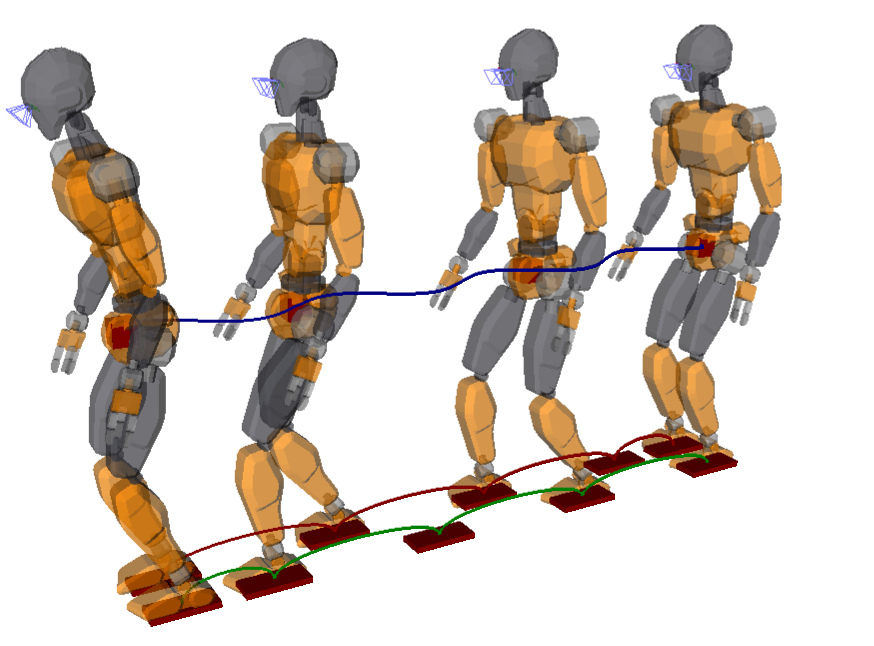Now our robot model is properly walking with a motion that is both kinematically and dynamically consistent. You can check out the complete walking controller in the horizontal_walking.py example in pymanoid.

## To go further

The concepts we have seen in this introduction are used in a full-fledged walking controller developed for walking and stair climbing with the HRP-4 humanoid.

The model predictive control problem we solved here to generate center of mass trajectories was reduced to the strict minimum: dynamic constraints on the ZMP and a terminal condition on the COM state. We also simplified things a bit by decoupling the x-axis and y-axis MPC, which is only valid when footstep edges are aligned with the axes of the inertial frame. You can find a more advanced implementation of this trajectory generation method in the ModelPredictiveControl.cpp source of the controller: it adds general footstep orientations, velocity and jerk terms to the cost function, as well as a (strict) terminal condition on a divergent component of motion. Those are implemented using the Copra linear MPC library.

There are two ways model predictive control has been applied to legged locomotion so far: open-loop and closed-loop MPC. In open-loop MPC is a motion planning approach where MPC outputs at the current step are fed to an integrator, which in turn determines MPC inputs for the next step. the plan is "unrolled" progressively

## Discussion

Thanks to all those who have contributed to the conversation so far. Feel free to leave a reply using the form below, or subscribe to the  Discussion's atom feed to stay tuned.

•Posted on

Thank you for your great explanation on WPG but I have a few questions regarding the LMPC formulation you used in this post. The states are composed of $$y$$, $$\dot{y}$$, and $$\ddot{y}$$ while the control input is the $$\dddot{y}$$ (COM jerk). In MPC control cycle, every state is measured first to provide initial states for each finite time horizon optimal control problem. My question is how you provide the $$\ddot{y}$$ to the LMPC (QP) solver. Do you just measure the $$\dot{y}(k)$$ and $$\dot{y}(k+1)$$ and use

$$\ddot{y}(k+1) = \frac{\dot{y}(k+1) - \dot{y}(k)}{T},$$

where $$T$$ is the discrete time step of MPC?

•Posted on

There are two main ways to use model predictive control in legged locomotion: open loop and closed loop MPC. Thank you for your question, which prompted the draft of this more detailed page :-) Open loop MPC is the approach we follow in this tutorial. We can see the integrator in run_com_mpc:

def run_com_mpc(self):
# ...
stance.com.integrate_constant_jerk(com_jerk, dt)
# ...


What this line does is, given the COM jerk $$\dddot{y}$$, compute the next state by forward integration:

\begin{align*} y(k + 1) & = y(k) + \dot{y}(k) T + \ddot{y}(k) {T}^2 / {2} + \dddot{y} {T}^3 / {6} \\ \dot{y}(k + 1) & = \dot{y}(k) + \ddot{y}(k) T + \dddot{y} {T}^2 / {2} \\ \ddot{y}(k + 1) & = \ddot{y}(k) + \dddot{y} T \end{align*}

If we measured velocities and computed accelerations by finite difference as you suggest, we would implement closed loop rather than open loop MPC. Both approaches have pros and cons, you can check out the linked page for more details and pointers.

You can use Markdown with $\LaTeX$ formulas in your comment.